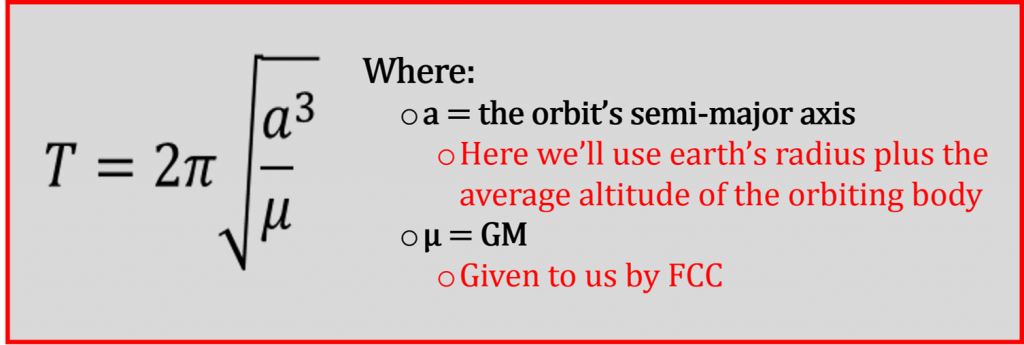## FCC Advanced Algorithm Scripting Challenge – ‘Map the Debris’

Today we’ll work through our first Free Code Camp advanced algorithm challenge called ‘Map the Debris‘, which will give us some exposure to working with objects.

This challenge asks us to ‘Return a new array that transforms the element’s average altitude into their orbital periods’

It further tells us that the array will contain objects in the following format:

Finally, it gives us the following as a starting point:

I think, given the limited amount that we’ve spent on objects here at crookedCode, we should start with a brief overview.

I’m not going to reinvent the wheel and write thousands of words on JavaScript objects when so many others have covered it much better than I ever could.

I will, however, point you to, and urge you to read, chapter 4 and chapter 6 of Eloquent JavaScript.  This will take some time, especially if you work through the examples (which I highly recommend) but you’ll have a much greater understanding of objects once you’re done.

Here, I’ll cover objects in enough detail to get us through this FCC algorithm.  If you’ve read the chapters mentioned above, this should all be review.

In JavaScript, objects are basically a collection of properties that can be wrapped together and stored in one variable. Properties consist of {key: value} pairs.

I think the best way to demonstrate this concept is through an example.  I don’t know why, but let’s say I wanted to create myself as an object…  It might look something like this:

This example shows 4 sets of key: value pairs, the first of which is – firstName: ‘Jeremy’ – with firstName being the ‘key’ and ‘Jeremy’ the ‘value’.  All 4 of these properties are now stored under the variable – me.

I can access and manipulate the properties through dot notation (bracket notation can also be used, but we’re going to stick with dot notation throughout this exercise).

In the example below I will access my age and change it to 21 (I know, I know, wishful thinking….).

As you can see when I logged the ‘me’ object to the console, the age has now been changed from 41 to 21.

We’ve seen object properties above as strings or numbers, but they can really be any data type, including other objects or functions.  When a property in an object is a function, it is said to be a ‘method’ of that function.  I’ll demonstrate how we can add new properties to objects below by giving ‘me’ a hair color property and a method.

Ok, suffice to say, this is only scratching the surface with objects in JavaScript, but I think we can move on to solving ‘Map the Debris’.

Before going any further I feel I should say there are many ways to solve this problem…  Some of them, I’m sure, are much more ‘slick’ than mine. This is the solution, however, that I wrote and am providing here as a working solution in an attempt to help anyone who is struggling or looking for a different perspective.

Solving ‘Map the Debris’

We know from the problem description that we will be getting an array of objects as an argument, and that each object in this array will have name and avgAlt properties.

We need to take each of the avgAlt properties, calculate the orbital periods (based on the equation given to us on the Wikipedia page referenced above), and return an array of objects that contain name and orbitalPeriod properties.

First things first, how do we calculate an orbital period?  Below is the formula given on Wikipedia.This equation might look a little intimidating if it’s been a while since your last math class, but we have all the elements we need.  We just have to rewrite the equation in JavaScript and fill in the correct values.

Fortunately, there’s a Math object in JavaScript that has all the methods associated with it that we’ll need (i.e. pi=Math.PI, squareroot=Math.sqrt(), exponents=Math.pow(base,exponent).  We’ll also use Math.round() to round our answer to the nearest whole number as required by the challenge.

My logic for setting up the solution was as follows:

• Write a function that solved the orbital period given the avgAlt (let’s call the function findOrbPeriod)
• Then write a loop to cycle through the array of objects that
• adds an orbitalPeriod property with a value calculated using the findOrbPeriod function.
• deletes the avgAlt property for each object in the array.
• Return the array.

If you’re working through Free Code Camp, I would suggest taking what we just talked about and try to solve the equation on your own.

Otherwise, proceed on…Let’s start by writing the function that will solve the orbital period.

As you can see, we use the avgAlt plus the earth’s radius for ‘axis’ in the equation.  The second line in the function is the orbital period equation listed above, only written using the JavaScript Math object.  Finally, we return the orbital period so we can use this function to populate the new orbitalPeriod property of each object.

Now we can tie everything together by cycling through the array of objects (arr) and calling the function we just wrote (findOrbPeriod()) for each object.

As you can see, the for loop adds an orbitalPeriod property to and removes the avgAlt property from each object in arr.  Then all we have to do is return arr.

There we go!!  We made it through our first  FCC advanced algorithm.  Hopefully this helped straighten out the code for you, feel free to leave any questions or comments below.  Until next time…

-Jeremy